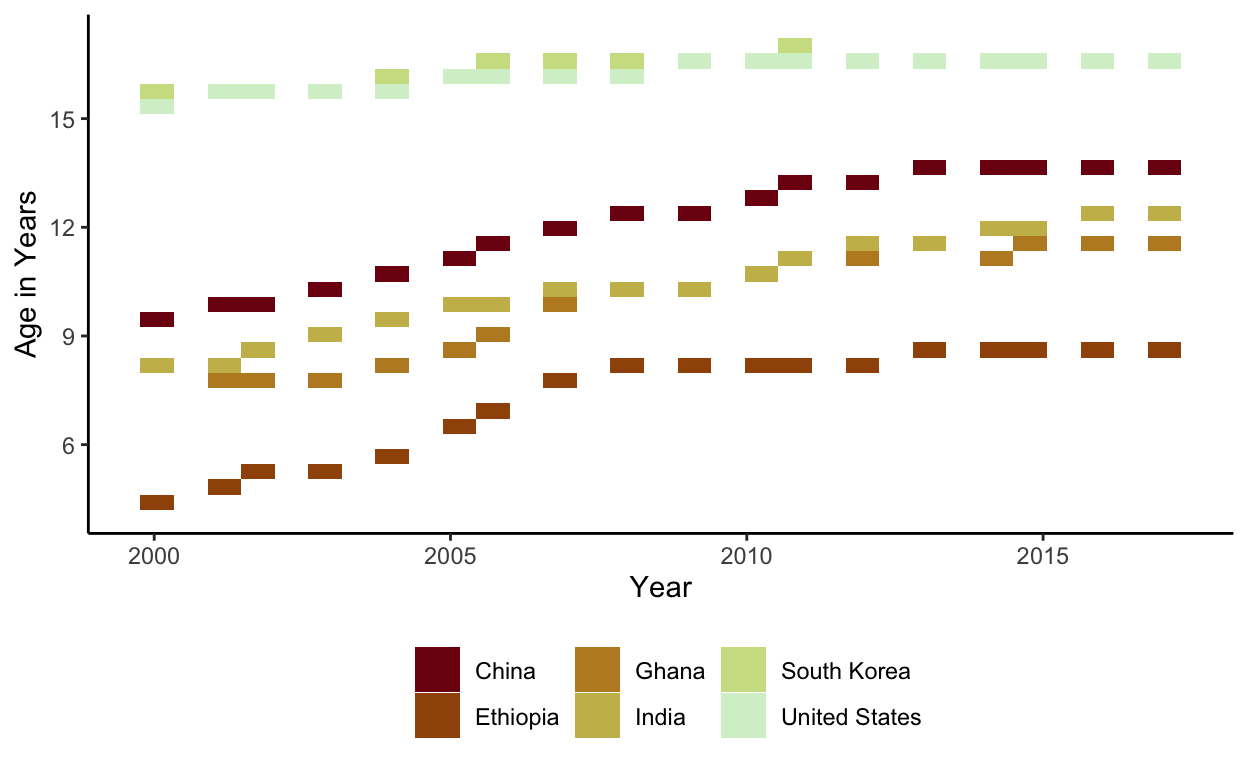# Project Part 2

Interactive and static plots for the age of students in school

1. Packages I will use to read in and plot the data
``````library(tidyverse)
library(echarts4r)
library(ggplot2)
``````
1. Read the data in from part 1
``````countries_age  <- read_csv(here::here("countries_age.csv"))
``````

# Interactive Graph

• Group_by country so there will be a “river” for each region
• Use mutate to round Age so only 2 digits will be displayed when you hover over it.
• Use mutate to change Year so it will be displayed as end of year instead of beginning of year
• Use e_charts to create an e_charts object with Year on the x axis
• Use e_river to build “rivers” that contain Age by countries. The depth of each river represents the age for each region.
• Use e_tooltip to add a tooltip that will display based on the axis values
• Use e_title to add a title, subtitle, and link to subtitle
• Use e_theme to change the theme to roma
``````countries_age   %>%
group_by(Country)  %>%
mutate(Age = round(Age, 2),
Year = paste(Year, "12", "31", sep="-"))  %>%
e_charts(x = Year)   %>%
e_river(serie = Age, legend=FALSE)  %>%
e_tooltip(trigger = "axis")  %>%
e_title(text = "Years in school by countries",
subtext = "(Age in Years). Source: Our World in Data",
left = "center")  %>%
e_theme("roma")
``````

# Static Graph

• Use ggplot to create a new ggplot object. Use aes to indicate that Year will be mapped to the x axis; Age will be mapped to the y axis; Country will be the fill variable
• geom_bin2d will display Age
• scale_fill_discrete_divergingx is a function in the colorspace package. It sets the color palette to roma and selects a maximum of 12 colors for the different regions
• theme_classic sets the theme
• theme(legend.position = “bottom”) puts the legend at the bottom of the plot
• labs sets the y axis label, fill = NULL indicates that the fill variable will not have the labelled Country
``````countries_age   %>%
ggplot(aes(x = Year, y = Age,
fill = Country)) +
geom_bin2d ()+
colorspace::scale_fill_discrete_divergingx(palette = "roma", nmax =11) +
theme_classic() +
theme(legend.position = "bottom") +
labs( y = "Age in Years",
fill = NULL)
``````This graph shows a small increase for the age of students in school since 2000.

``````ggsave(filename = here::here("_posts/2022-05-16-project-part-2/preview.png"))
``````